We will often use the Align component in our development to position the child components. Usually we use several common relative positions, such as alignment: Alignment.bottomRight, bottom-right corner. Recently, the official documentation about alignment: Alignment(x, y) in Align seems to be a little bit wrong, and then combined with the relevant source code, we finally figured it out. Then I found that most of the tutorials on the Internet are wrong, so I’ll share my understanding of Align’s alignment principle below.

## Pre-requisite knowledge: Alignment

Alignment works by first creating a virtual coordinate system in a rectangle. The origin of this virtual coordinate system is the midpoint of the rectangle, then (x, y) is the locus in this virtual coordinate system, and the values of (x, y) are specified to represent relative values. For example, Alignment(0.0, 0.0) is the midpoint and Alignment(1.0, 1.0) is the lower right corner, as shown in the figure.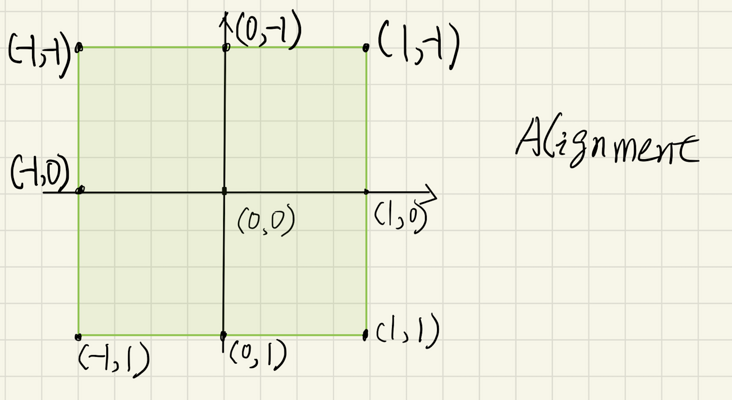The unit of the relative value of x in (x, y) is half of the width of the rectangle, for example, 2.0 is equivalent to the entire width of the rectangle; y is similar, the unit of y is half of the height of the rectangle.

This is then converted to a point of orientation in the coordinate system with the upper left corner of the rectangle as the origin by a formula. This conversion formula is as follows: `(x * w/2 + w/2, y * h/2 + h/2)`, where w is the width of the rectangle and h is the height of the rectangle. The calculation process is understood in conjunction with the following schematic diagram.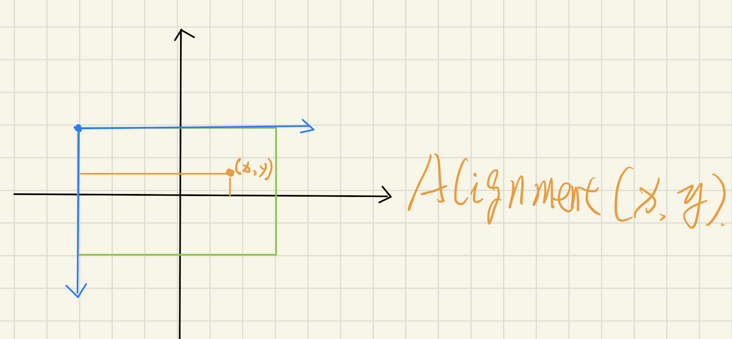The green box is this rectangle, the black axis is the virtual coordinate system, and the blue axis is the target coordinate system with the upper left corner of the rectangle as the origin. Taking the horizontal coordinate x on the virtual coordinate axis as an example, the converted target point’s horizontal coordinate x’ = half of the rectangle width plus x * half of the rectangle width (the unit of x in the virtual coordinate axis is half of the rectangle width).

For example, the value of Alignment(2.0, 0.0) is 1.5 times the width of the rectangle, not twice the width of the rectangle.

## How Align works

In the official Align documentation there is this sentence.

How it works

The alignment property describes a point in the child’s coordinate system and a different point in the coordinate system of this widget. The Align widget positions the child such that both points are lined up on top of each other.

## Misunderstanding

From the above description, we can see that the final placement coordinates are related to the size of Align as well, because the process uses alignment to calculate a point in Align. But I only see from the official documentation that the calculation is related to the subcomponent.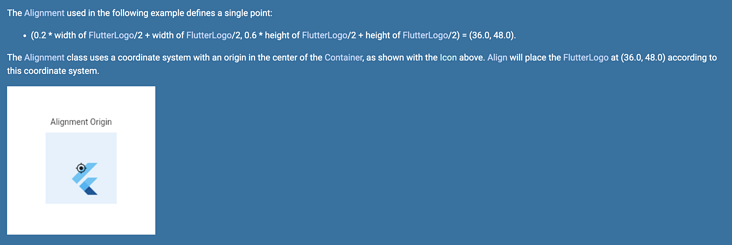And some of the tutorials I’ve seen online have explanations that are wrong, such as this one.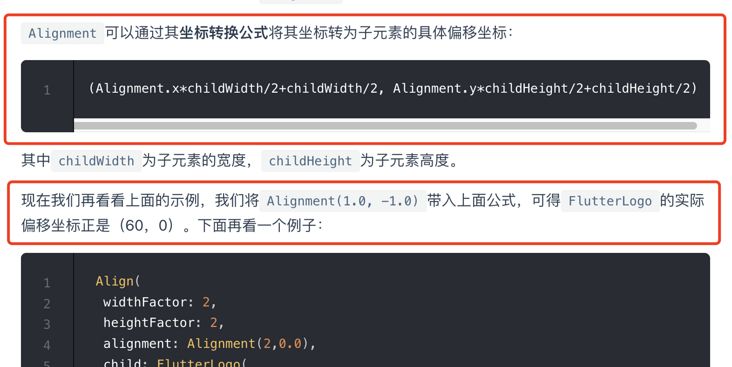To prove this error is simple, place Align in a Container and set alignment to Alignment(1.0, 0.0). Then place a random child component, like this.

 `````` 1 2 3 4 5 6 7 8 9 10 11 12 13 `````` ``````Container( height: 120, width: 200, color: Colors.green.withOpacity(0.6), child: Align( alignment: Alignment(1.0, 0.0), child: Container( width: 60, height: 60, color: Colors.red, ), ), ), ``````

You will notice that no matter how you change the width of the outside Container, the right side of the red rectangle inside always coincides with the right side of the outside Container. How does this work if the coordinates of the positioning are only related to the width of the child component?

## My understanding

The official documentation on how it works gives the principle of the calculation, but not the exact formula. I’ve looked at the source code to confirm that the description of how it works is fine, so I’ll use a simple example to illustrate the calculation process and give the correct formula.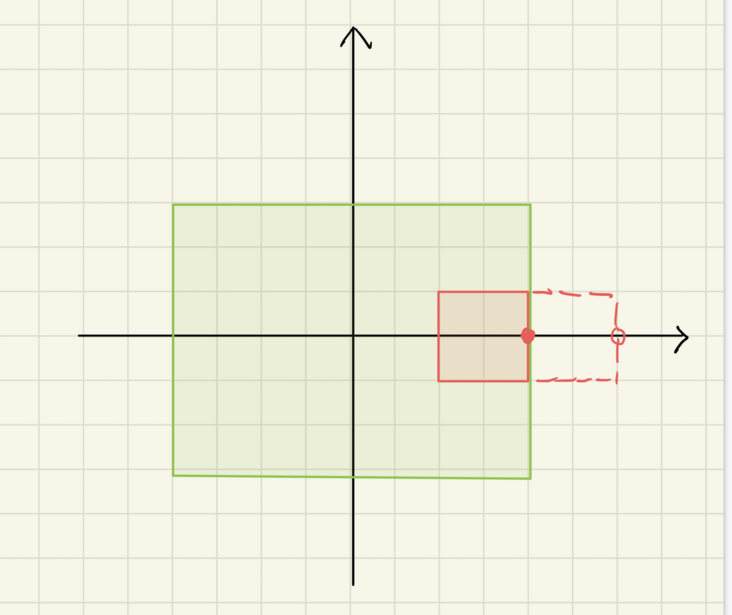The green rectangle above is the size of Align, the red is the subcomponent, and I’ll use Alignment(1.0, 0.0) to illustrate this. the horizontal coordinate of the point described by Alignment(1.0, 0.0) is at the right box in both the green and red rectangles, and if Align positions the subcomponent according to this position, the red rectangle should be at the red dotted box If Align had positioned the subcomponent in this way, the red rectangle would be in the red dotted box. However, according to the description of how it works, Align places the subcomponent so that the two points coincide, i.e. the point on the right side of the red rectangle coincides with the point on the right side of the green rectangle. In this case, we just need to move from the position of the red dotted box to the position of the solid box, i.e., the horizontal coordinate of the point positioned in Align minus the horizontal coordinate of the point positioned in the subcomponent.

 ``````1 `````` ``````alignWidth / 2 + x * (alignWidth / 2) - childWidth / 2 - x * (childWidth / 2) ``````

A similar process can be described for the y-values of the coordinates, so the formula for the final locus should be

 ``````1 2 `````` ``````var x = (alignWidth - childWidth) / 2 + x * ((alignWidth - childWidth) / 2); var y = (alignHeight - childHeight) / 2 + x * ((parentHeight - childHeight) / 2); ``````

Finally, if alignment is FractionalOffset, a similar process can be used to describe positioning. The difference between FractionalOffset and Alignment is that the origin of FractionalOffset in the virtual coordinate system is in the upper left corner of the rectangle, and the unit of x in FractionalOffset(x, y) is the entire width of the rectangle, not half. Positioning in Align still follows that the points of the two FractionalOffsets should be coincident.

The difference between FractionalOffset and Alignment is that the origin of FractionalOffset in the virtual coordinate system is in the upper-left corner of the rectangle, and the unit of x in FractionalOffset(x, y) is the full width of the rectangle, not half of it. Positioning in Align still follows that the points of the two FractionalOffsets should be coincident.